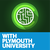## Logical Operators Misunderstanding

I've got to ask, tried looking online not much luck, could someone talk through logical operators? like questions in a quiz was find u when u = 5<<2 or u = 9|1 and u=5^5 i get that << is shift in binary but when the numbers get to 100 or 76 how would you figure it out without spending ages looking at the binary values sheet? and also is ^ just arithmetic subtraction? and don't understand too much about | i get that is a NOT and flips the binary value but can someone explain in more detail please?

#### Question relating to:STUDENTS Team School of Computing Electronics and Mathematics Plymouth University UK

##### 5 years, 3 months ago.

See below

Q. I've got to ask, tried looking online not much luck, could someone talk through logical operators? like questions in a quiz was find u when u = 5<<2

Tutors Answer: A left shift by 2 is equivalent to multiply by 4

Q. or u = 9|1 and u=5^5 i get that << is shift in binary but when the numbers get to 100 or 76 how would you figure it out without spending ages looking at the binary values sheet?

Tutors Answer: most calculators can convert numbers to and from binary, including the calculator applications in Windows and OS X. Also, you could write some code to perform the calculations!

and also is ^ just arithmetic subtraction?

Tutors Answer: NO - it is exclusive OR - see the lectures slides

and don't understand too much about | i get that is a NOT and flips the binary value but can someone explain in more detail please?

See one of us in the lab and we can explain.